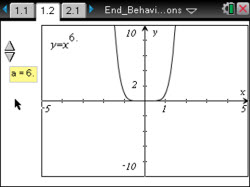# Activities

•##### Download

•• ##### Subject Area

• Math: Precalculus: Polynomial, Power, and Rational Functions

• ##### Author9-12

45 Minutes

• ##### Device
•TI-Nspire™ CX/CX II
•TI-Nspire™ CX CAS/CX II CAS
• TI-Nspire™ Navigator™
• TI-Nspire™
• TI-Nspire™ CAS
• ##### Software

TI-Nspire™
TI-Nspire™ CAS

3.0

• ##### Report an Issue

End Behavior of Polynomial Functions#### Activity Overview

This lesson involves determining the similarities and differences among functions.

#### Objectives

• Students will recognize the similarities and differences among power and polynomial functions of various degrees.
• Students will describe the effects of changes in the leading coefficient on the end behavior of graphs of power and polynomial functions.

#### Vocabulary

• degree of a polynomial
• end behavior
• leading coefficient
• polynomial function
• power function

#### About the Lesson

This lesson involves determining the similarities and differences among functions.
As a result, students will:

• Use a slider to scroll through the graphs of power functions with a coefficient of positive 1 and graphs of power functions with a coefficient of negative 1.
• Generalize the end-behavior properties of various power and polynomial functions.
• Write a possible equation of a polynomial function given the graph of the function.